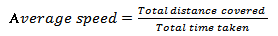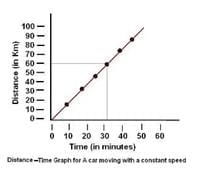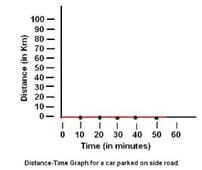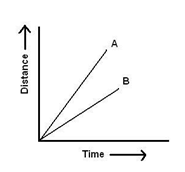# Short & Long Question Answers: Motion & Time Notes | Study Science Class 7 - Class 7

## Class 7: Short & Long Question Answers: Motion & Time Notes | Study Science Class 7 - Class 7

The document Short & Long Question Answers: Motion & Time Notes | Study Science Class 7 - Class 7 is a part of the Class 7 Course Science Class 7.
All you need of Class 7 at this link: Class 7

Short Q & A :

Q1: What are the points that should be kept in mind while choosing scale for drawing graph?

Ans :

The following points should be kept in mind while choosing most suitable scale for drawing a graph

• The difference between the highest and the lowest values of each quantity.
• The intermediate values of each quantity to mark the values on the graph.
• To utilize the maximum part of the paper on which graph is to be drawn.

Q2: What is the advantage of a distance- time graph?

Ans : Distance-time graphs gives information about the nature of the motion of an object like uniform or uniform motion. Motion of an object can be represented by its distance-time graphs.

Q3: Differentiate between distance and displacement.

Ans : The distance covered by a moving object is the actual length of the path followed by the object is called. Distance is a scalar quantity. SI unit of distance is meter. But Displacement is the shortest distance covered by a moving object from the point of reference (initial position of the body), in a specified direction.

Q4: Differentiate between uniform speed and average speed.

Ans : An object is said to be moving with uniform speed if it covers equal distances in equal intervals of time. But when we travel in a vehicle the speed of the vehicle changes from time to time depending upon the conditions existing on the road. In such a situation, the speed is calculated by taking the ratio of the total distance travelled by the vehicle to the total time taken for the journey. This is called the average speed.

Q5: What do you mean by non-uniform speed?

Ans : An object is said to be moving with variable speed or non-uniform speed if it covers equal distances in unequal intervals of time or vice-versa.

Q6: What do you mean by instantaneous speed?

Ans : When we say that the car travels at an average speed of 60 km/h it does not mean that the car would be moving with the speed of 60 km/h throughout the journey. The actual speed of the car may be less than or greater than the average speed at a particular instant of time. The speed of a moving body at any particular instant of time is called instantaneous speed.

Q7: A child is on see-saw, what kind of motion he have and why? Explain.

Ans : On a see-saw child goes up and comes down from mean position and repeats itself .So there is oscillatory motion.

Q8: Explain how in ancient time a day, a month and a year were measured.

Ans : In ancient time the time between one sunrise and the next was called a day. A month was measured from one new moon to the next and a year was fixed as the time taken by the earth to complete one revolution of the sun.

Q9: Define velocity along with its unit.

Ans : Velocity can be defined as the rate of change of displacement.SI unit of velocity is m/s (meter/second Velocity is a vector quantity.

Q10: What are quartz clocks?

Ans : It is a special type of clock or watch which have an electric circuit with one or more cells are called quartz clocks.

Q11: How do we know an object is moving faster compared to the speed of another object?

Ans : By finding the distance travel by a moving body in unit time 1 hr. or 1 sec we know the speed of an object that help us to know which one is moving faster i.e. having greater speed.

Q12: A simple pendulum takes 15 seconds to complete 5 oscillations. What is the time period of pendulum?

Ans :

The time taken by pendulum to complete 1 oscillation is called time period

The time taken by pendulum to complete 5 oscillation = 15sec

The time taken by pendulum to complete 1 oscillation = 15/5= 3 sec

So time period = 3 sec

Q13: If a car is moving with a speed of 5Km/h on highway then find the distance travelled by the car in 4 hours?

Ans :

We know distance travelled by a body = speed X time

So, distance travelled by the car =5x4=20 km

Q14: Sumit covers a distance of 2.4 Km from his house to reach her college on a scooter. If the scooter has a speed of 6m/sec, calculate the time taken by her to reach the college.

Ans :

Total distance travelled =2.4km=2.4x1000m=2400m. Speed is 6m/sec

as time = (Distance/speed) time=2400/6= 400sec.

Q15: How can you say that motion and rest are relative?

Ans : We have observed that the position of stars and planets change while you remain stationary. In reality the earth is moving too. Thus, an object which appears to be at rest, may actually be in motion. Therefore, motion and rest are relative terms.

Q16: Differentiate between circular motion and periodic motion.

Ans : Motion of an object in a circular path is called circular motion eg. Motion of the hands of a clock. But when an object repeats its motion after some time. This type of motion is called periodic motion. E.g. Motion of a pendulum, motion of a child on a swing, motion of the strings of a guitar.

Q17: Give an example when objects undergo combinations of different types of motion?

Ans : The motion of a ball on the ground. Here the ball is rolling on the ground and rotating as well as moving forward on the ground. Thus, the ball undergoes a rectilinear motion as well as rotational motion.Q18: What is simple pendulum?

Ans : A simple pendulum consists of a small metallic ball or a piece of stone suspended from a rigid stand by a thread .The metallic ball is called the bob of the pendulum.

Q19: What is oscillation of a pendulum?

Ans : One complete to and fro motion of a pendulum from rest position is called one oscillation. The time taken by the pendulum to complete one oscillation is called its time period. It is only depending on the length of the string of pendulum.

Q20: State different types of motion?

Ans :

Following are different types of motion:

• Translatory Motion: - In Translatory motion the particle moves from one point in space to another. This motion may be along a straight line or along a curved path.
• Rectilinear motion : Motion along a straight line is called rectilinear motion. Example: A car moving on a straight road
• Curvilinear motion: Motion along a curved path is called curvilinear motion. Example: A car negotiating a curve
• Rotatory Motion : In rotatory motion, the particles of the body describe concentric circles about the axis of motion
• Vibratory Motion: In vibratory motion the particles move to and fro about a fixed point.

Q21: What do you mean by time?

Ans : The interval between two events is called time. Example: The time between one sunrise and the next was called a day. Clocks or watches are the most common time measuring devices. The basic unit of time is a second. Its symbol is s. Larger units of time are minutes (min) and hours (h).

Q22: Explain how we can plot a graph.

Ans :

To plot a graph on a graph sheet:

-Take a graph paper.

-At the centre of the paper, draw two lines perpendicular to each other Mark the point of intersection of the lines as ‘O. The horizontal line is called the X-axis, while the vertical line is called the Y-axis. The point of intersection of the two axes is called the origin, "O". Take one quantity along the X-axis and the other along the Y-axis, after choosing suitable scales for both. For every value along one axis, mark a point matching the related value on the other axis. Join the points, and your graph is ready.

Q23: A simple pendulum takes 32 s to complete 20 oscillations. What is the time period of the pendulum?

Ans :

Time taken to complete 20 oscillations = 32 s

Time period of the pendulum = No of oscillations/Total Time taken = 32/20 s = 1.6s

Q24: The odometer of a car reads 57321.0 km when the clock shows the time 08:30 AM. What is the distance moved by the car, if at 08:50 AM, the odometer reading has changed to 57336.0 km? Calculate the speed of the car in km/min during this time. Express the speed in km/h also.

Ans :

Initial reading of car odometer= 57321.0 km

Final reading of car odometer= 57336.0 km

Time at the time of Initial reading= 08:30 AM

Time at the time of Initial reading= 08:50 AM

Distance travelled by the car = 57336.0 km - 57321.0 km

= 15 Km

Time taken by the car to cover distance = 08:50 AM -08:30 AM

= 20 m

Speed of the car in Km/m = 15 km/20m

= .75 Km/m

Speed of the car in Km/h = 15 km/20m X 60

= 45 Km/h

Q25: Salma takes 15 minutes from her house to reach her school on a bicycle. If the bicycle has a speed of 2 m/s, calculate the distance between her house and the school.

Ans :

Distance between her school and house = speed of bicycle X Time taken

= 2m/s × 60s= 1800m

Q26: The distance between two stations is 300 km. A train takes 6 hours to cover this distance. Calculate the speed of the train.

Ans :

The distance between two stations = 300 km

Time taken by the train to cover that distance = 6 hours

Speed of the train = 300/6 Km/h =50 km/h

Q27: Show the shape of the distance-time graph for the motion in the following cases when a car is moving with a constant speed.

Ans :Q28: Show the shape of the distance-time graph for the motion when a car parked on a side road.

Ans :Q29: Look at the graph below of two vehicles A and B, which one of them is moving faster.Ans :

Q30: A car moves with a speed of 40 km/h for 5 minutes and then with a speed of 60 km/h for the next 5 minutes. The total distance covered by the car is:

Ans :

Distance covered by the car with a speed

of 40 km/h in 15 minutes = Speed * Time

= (40 Km/h /60 minute) * 15 minute

Distance covered by the car with a speed

of 40 km/h in 15 minutes = Speed * Time

= (60 Km/h /60 minute) * 15 minute

= 15 Km

Total distance covered by the car =(10 + 15 )km = 25 Km

Long Q & A :

Q1: Classify the following as motion along a straight line, circular or oscillatory motion:

Motion of our hands while running

Motion of a horse pulling a cart on a straight road.

Motion of a kid in a merry-go-round.

Motion of a child on a see-saw.

Motion of the hammer of an electric bell.

Motion of a train on a straight bridge

Ans :

Motion of our hands while running. : - oscillatory motion

Motion of a horse pulling a cart on a straight road. :- straight line motion

Motion of a kid in a merry-go-round. : - straight line motion

Motion of a child on a see-saw. : - oscillatory motion

Motion of the hammer of an electric bell.- oscillatory motion

Motion of a train on a straight bridge : - straight line motion

The document Short & Long Question Answers: Motion & Time Notes | Study Science Class 7 - Class 7 is a part of the Class 7 Course Science Class 7.
All you need of Class 7 at this link: Class 7Use Code STAYHOME200 and get INR 200 additional OFF

## Science Class 7

161 videos|216 docs|54 tests

Track your progress, build streaks, highlight & save important lessons and more!

,

,

,

,

,

,

,

,

,

,

,

,

,

,

,

,

,

,

,

,

,

;